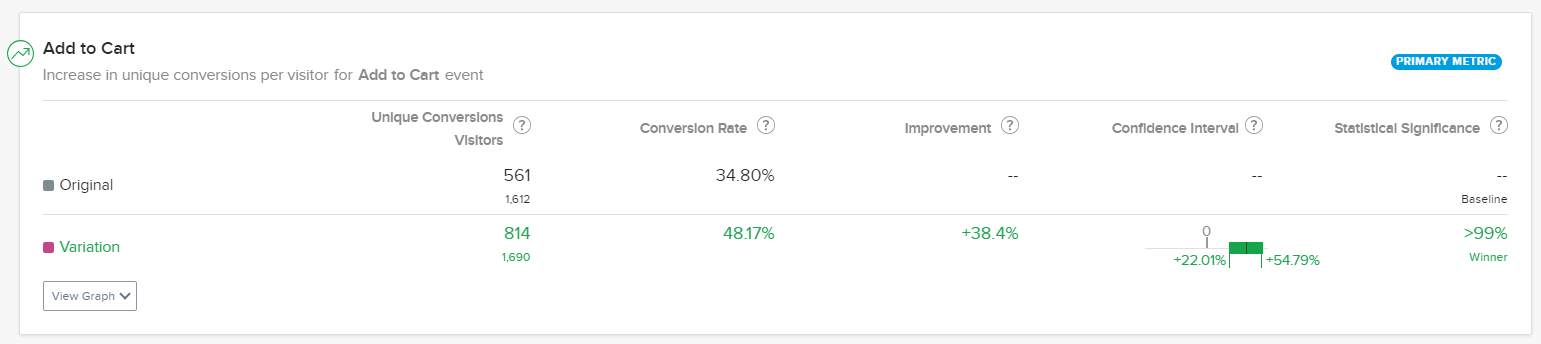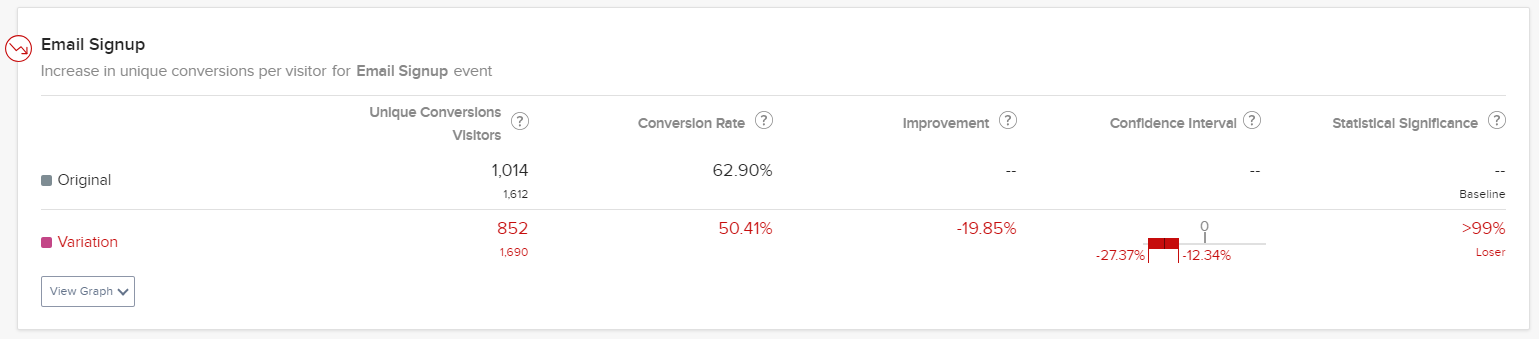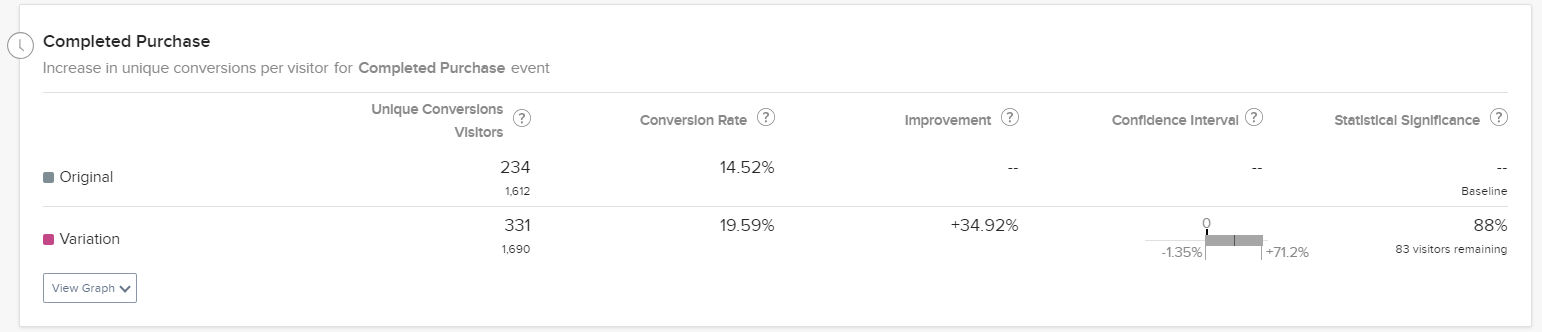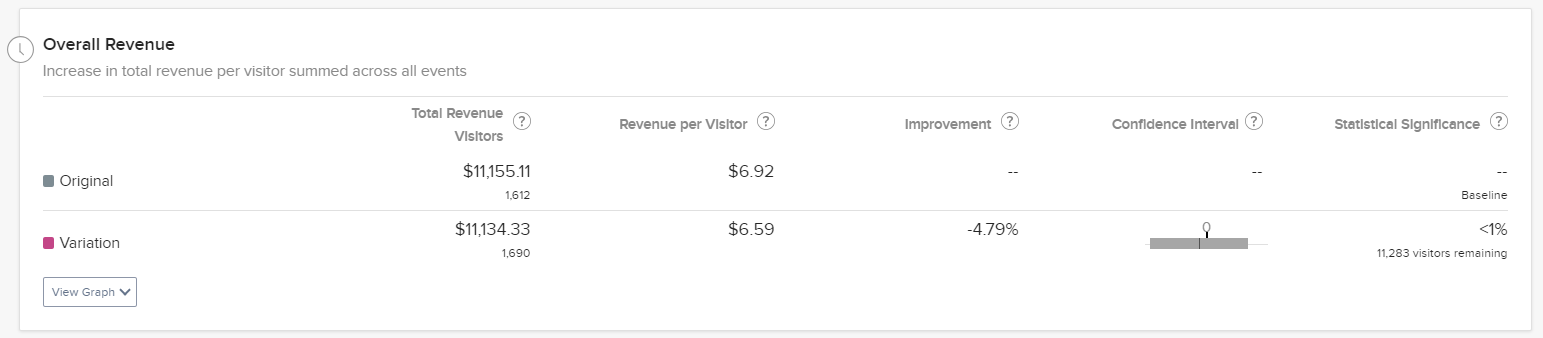# Confidence intervals and improvement intervals

• Updated

This topic describes how to:

• Use confidence intervals and improvement intervals to analyze results
• Predict what behavior you should see from your results over time

Statistical significance tells you whether a variation is outperforming or underperforming the baseline, at whatever confidence level you chose. Confidence intervals tell you the uncertainty around improvement. Stats Engine provides a range of values where the conversion rate for a particular experience lies. It starts wide, but as Stats Engine collects more data, the interval narrows to show that certainty increases.

Optimizely Experimentation sets your confidence interval at the same level that you put your statistical significance threshold for the project. The statistical significance setting for your project is 90% by default.

A variation is declared significant as soon as the confidence interval stops crossing zero. Intervals crossing zero mean there is not enough evidence to say if there is a clear impact or not.

Once a variation reaches statistical significance, the confidence interval always lies entirely above or below 0.

• A winning variation will have a confidence interval that is entirely above 0%.

• An inconclusive variation will have a confidence interval that includes 0%.

• A losing variation will have a confidence interval that is entirely below 0%.

## Example: Winning intervalIn the example shown above, you can say there is a 99% chance assuming your variation has zero impact on influencing the behavior of your user population compared to your baseline. You would observe a lift that large or larger in 1% of your experiments due to random fluke.

But the improvement Optimizely Experimentation measured (+38.4%) may not be the exact improvement you see going forward.

In reality, if you implement that variation instead of the original, the relative improvement in conversion rate will probably be between 22.01% and 54.79% over the baseline conversion rate. Compared to a baseline conversion rate of 34.80%, you are likely to see your variation convert between 42.46% (34.80 + 34.80*0.2201) and 53.87% (34.80 + 34.80*0.5479).

The statistical significance setting for your project is 90% which can tell you whether the results are consistent with being due to chance. As Optimizely Experimentation collects more data, the confidence interval may narrow.

## Example: Poor Performance

This example shows the time with the confidence interval entirely below 0.In this example, you can say that there is a 99% chance that the negative improvement you saw in the bottom variation is not due to chance. The improvement Optimizely Experimentation measured (-19.85%) is likely to be what you see going forward.

In reality, the difference in conversion rate will probably be between -27.37% and -12.34% under the baseline conversion rate if you implement the variation instead of the original. Compared to a baseline conversion rate of 62.90%, you are likely to see your variation convert between 45.68% (62.90 - 62.90*0.2737) and 55.14% (62.90 - 62.90*0.1234).

In this experiment, the observed difference between the original (62.90%) and variation (50.41%) was -12.49%, just within the confidence interval. If we rerun this experiment, the baseline and variation conversion rate difference will probably be in the same range.

## Example: Inconclusive interval

Suppose you must stop a test early or have a low sample size. In that case, the confidence interval will give you a rough idea of whether implementing that variation will have a positive or negative impact.

For this reason, when you see low statistical significance on specific goals, the confidence interval can serve as another data point to help you make decisions. When you have an inconclusive goal, the interval will look like this:Here, we can say that this variation's conversion rates will be between -1.35% and 71.20%. You can interpret the confidence interval as a worst-case, middle-ground, and best-case scenario. For example, we are 90% confident that the worst-case difference between variation and baseline conversion rates is -1.35%, the best case is 71.20%, and a middle ground is 34.93%.

## How statistical significance and confidence intervals are connected

Optimizely Experimentation shows you the statistical likelihood that the improvement is due to changes you made on the page, not chance. Until Stats Engine has enough data to declare statistical significance, the Results page will state that more visitors are needed and show you an estimated wait time based on the current conversion rate.

Lower significance levels may increase the likelihood of error but can also help you test more hypotheses and iterate faster. Higher significance levels decrease the error probability but require a larger sample.

Choosing the right significance level should balance the types of tests you are running, the confidence you want to have in the tests and the amount of traffic you receive.

Significance and confidence intervals are connected. Your experiment reaches significance at precisely the same time your confidence interval on improvement moves away from zero.

## Improvement intervals

Depending on what type of experiment or optimization you are running, Optimizely Experimentation will display a different improvement interval on the Results page.

• For A/B experiments – Optimizely Experimentation displays the relative improvement in conversion rate for the variation over the baseline as a percentage for A/B experiments. This is true for all A/B experiment metrics, regardless of whether they are binary conversions or numeric.
• In Optimizely Experimentation, a relative improvement interval of 1% to 10% means that the variation sees between 1% and 10% improvement over the baseline. For example, if the baseline conversion rate is 25%, you can expect the variation conversion rate to fall between 25.25% and 27.5%.
• For Multi-armed Bandit (MAB) optimizations – Optimizely Experimentation displays absolute improvement.
• For A/B experiments or MAB optimizations with Stats Accelerator enabled – Optimizely Experimentation displays the absolute improvement.
MAB optimizations do not generate statistical significance.

## Estimated wait time and <1% significance

As your experiment or campaign runs, Optimizely Experimentation estimates how long it will take for a test to reach conclusiveness.

This estimate is based on the current observed baseline and variation conversion rates. If those rates change, the estimate will adjust automatically.You may see a significance of less than 1%, with a certain number of "visitors remaining." What does this mean? In statistical terms, this experiment is underpowered: Optimizely Experimentation needs to gather more evidence to determine whether the change you see is a true difference in visitor behaviors or chance.

Look at the variation in the example shown above. Optimizely Experimentation needs approximately 11,283 more visitors to be exposed to that variation before deciding the conversion rates between the variation and the original. Remember that the estimated 11,283 visitors assumes that the observed conversion rate does not fluctuate. If more visitors see the variation but conversions decrease, your experiment will probably take more time, which means the "visitors remaining" estimate will increase. If conversions increase, Optimizely Experimentation will need fewer visitors to be sure that the behavior change is real.

To learn more about the importance of sample size, see our article on how long to run a test.

Unlike many testing tools, Optimizely Experimentation's stats engine uses a statistical approach that removes the need to decide on sample size and minimum detectable effect (MDE) before starting a test. You do not have to commit to large sample sizes ahead of time, and you can check on results whenever you want!

However, many optimization programs estimate how long tests take to run so they can build robust roadmaps. Use our sample size calculator to estimate the number of visitors you need for a test. Learn more about choosing a minimum detectable effect for our calculator in using the minimum detectable effect to prioritize experiments.

<% if (previousArticle || nextArticle) { %>
<% if (previousArticle) { %>
<% if (previousTitle) { %>

#### <%= previousTitle %>

<% } %> <%= previousArticle.title %>
<% } %> <% if (nextArticle) { %>
<% if (nextTitle) { %>

#### <%= nextTitle %>

<% } %> <%= nextArticle.title %>
<% } %>
<% } %>
<% if (allItems.length > 1) { %>